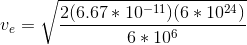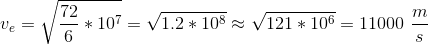# AP Physics C: Mechanics : Potential Energy

## Example Questions

### Example Question #5 : Energy

Calculate how much potential energy a vertical standing spring gains if someone puts a 4kg box on top of it. Take the spring constant to be 120N/s.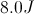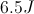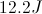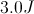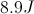Explanation:

First, find out how much the spring compresses when the 4kg box is put on top of it. To find this, consider what forces are acting on the box when it is resting on the spring. The forces acting on it are the upward spring force,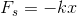, and the downward gravitational force,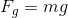; thus, the net force acting on the box is given by the equation below.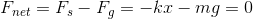We have chosen the upward direction to be positive and the downward direction to be negative. The net force is equal to zero because the box is at rest. Solve for x.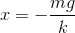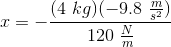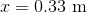Then use this value to find the potential energy of the spring.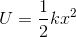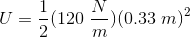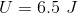So when the box is placed on top of the spring, the spring gains a potential energy of 6.5J.

### Example Question #161 : Mechanics Exam

A man throws a 5kg ball straight up. At the top of its trajectory, the ball has a potential energy of 160 Joules. What is the highest point the ball reaches if it is thrown from the level of the ground?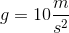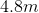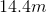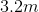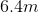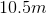Explanation:

The equation for potential energy is: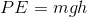We can rearrange to solve for the height: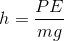Plug in our given values to solve: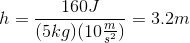### Example Question #162 : Mechanics Exam

A man throws a 5kg ball straight up. At the top of its trajectory, the ball has a potential energy of 160 Joules. At what velocity does the man initially throw the ball?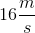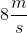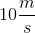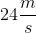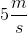Explanation:

We can use conservation of energy to find the velocity required to launch the ball.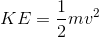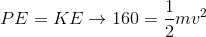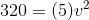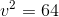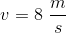### Example Question #11 : Energy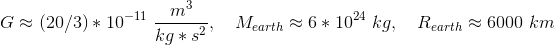A man launches a 5kg ball straight up from sea level. Disregarding drag, if the man would like to launch the ball into an escape trajectory from earth's gravity, approximately how fast must he launch the ball (in meters per second)?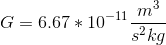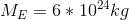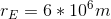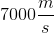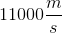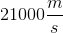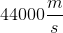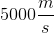Explanation:

In order to launch the ball at a velocity that escapes Earth's gravity, the kinetic energy must overcome the gravitational pull of the Earth. We can use conservation of energy to make the kinetic energy and gravitational potential energies equivalent.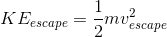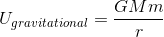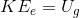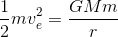Rearrange to solve for the escape velocity: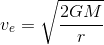Finally, plug in our values and solve.